# Class 10 RD Sharma Solutions – Chapter 4 Triangles – Exercise 4.3

• Last Updated : 08 Dec, 2020

### (i) If BD = 2.5 cm, AB = 5 cm and AC = 4.2 cm, find DC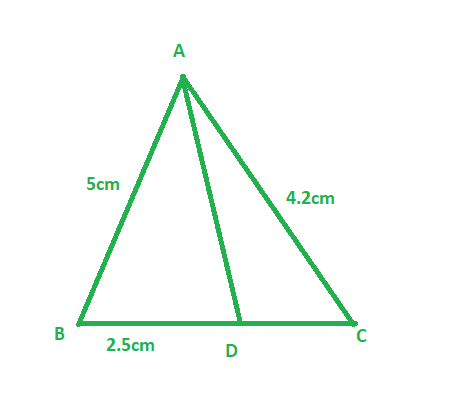Solution:

Hey! Looking for some great resources suitable for young ones? You've come to the right place. Check out our self-paced courses designed for students of grades I-XII

Start with topics like Python, HTML, ML, and learn to make some games and apps all with the help of our expertly designed content! So students worry no more, because GeeksforGeeks School is now here!

Given:

Length of side BD = 2.5 cm, AB = 5 cm, and AC = 4.2 cm.

To find: Length of side DC

In Δ ABC, AD is the bisector of ∠A, meeting side BC at D.

Therefore,

AB/AC = 2.5/DC

5/4.2 = 2.5/DC                 ( Since, AB = 5 cm, and AC = 4.2 cm )

5DC = 4.2 × 2.5

DC = (4.2 × 2.5)/5

DC = 2.1cm

Therefore, Length of side DC is 2.1 cm

### (ii) If BD = 2 cm, AB = 5 cm and DC = 3 cm, find AC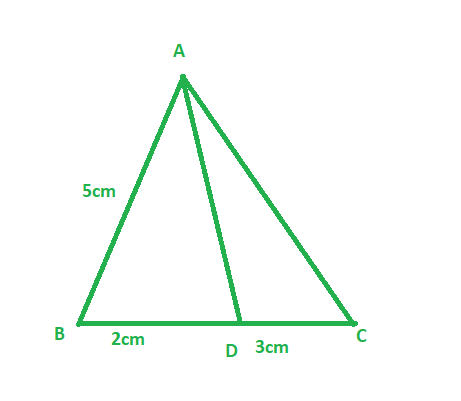Solution:

Given:

Length of side BD = 2 cm, AB = 5 cm, and DC = 3 cm

To find: Length of side AC

In Δ ABC, AD is the bisector of ∠A, meeting side BC at D

Therefore,

AB/AC = BD/DC                                                (since AD is the bisector of ∠A and side BC)

5/ AC = 2/3                                                     (Since, BD = 2 cm, AB = 5 cm, and DC = 3 cm )

2AC = 5 × 3

AC = 15/2

AC = 7.5 cm

Therefore ,Length of side AC is 7.5 cm

### (iii) If AB = 3.5 cm, AC = 4.2 cm and DC = 2.8 cm, find BD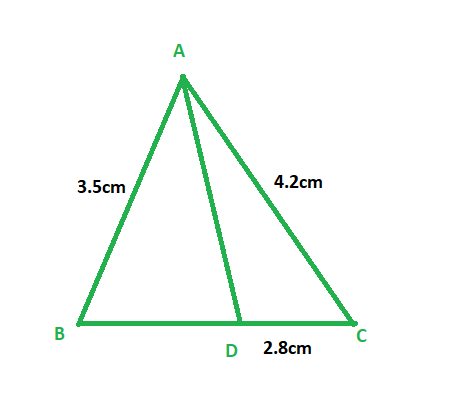Solution:

Given:

Length of side AB = 3.5 cm, AC = 4.2 cm, and DC = 2.8 cm

To find: Length of side BD

In Δ ABC, AD is the bisector of ∠A, meeting side BC at D

Therefore,

⇒ AB/ AC = BD/ DC

3.5/ 4.2 = BD/ 2.8                       (Since, AB = 3.5 cm, AC = 4.2 cm, and DC = 2.8 cm)

4.2 x BD = 3.5 × 2.8

BD = 7/3

∴ BD = 2.3 cm

Therefore, Length of side BD is 2.3 cm

### (iv) If AB = 10 cm, AC = 14 cm and BC = 6 cm, find BD and DC.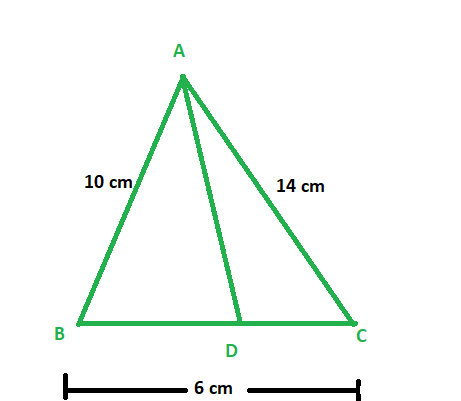Solution:

Given:

Length of side AB = 10 cm, AC = 14 cm, and BC = 6 cm

To find: Length of side BD and DC

In Δ ABC, AD is the bisector of ∠A meeting side BC at D

Since, AD is bisector of ∠A

Therefore,

AB/AC = BD/DC                    – equation 1

Let BD be x, then DC = 6-x

Now, putting values in equation 1

⇒10/ 14 = x/ (6 – x)

14x = 60 – 6x

20x = 60

x = 60/20

∴ BD = 3 cm and DC = (6 – 3) = 3 cm.

Therefore, Length of side BD is 3 cm and DC is 3cm

### (v) If AC = 4.2 cm, DC = 6 cm and BC = 10 cm, find AB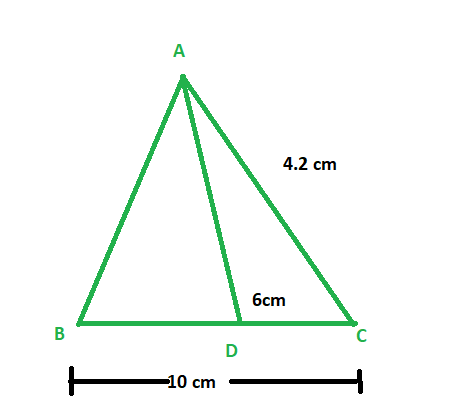Solution:

Given:

Length of side AC = 4.2 cm, DC = 6 cm, and BC = 10 cm.

To find: Length of side AB

In Δ ABC, AD is the bisector of ∠A, meeting side BC at D.

Since, AD is the bisector of ∠A

Therefore, we get

⇒ AB/ AC = BD/ DC

AB/ 4.2 = BD/ 6

We know that,

BD = BC – DC = 10 – 6 = 4 cm

⇒ AB/ 4.2 = 4/ 6

AB = (2 × 4.2)/ 3

∴ AB = 2.8 cm

Therefore, Length of side AB is 2.8 cm

### (vi) If AB = 5.6 cm, AC = 6 cm and DC = 3 cm, find BC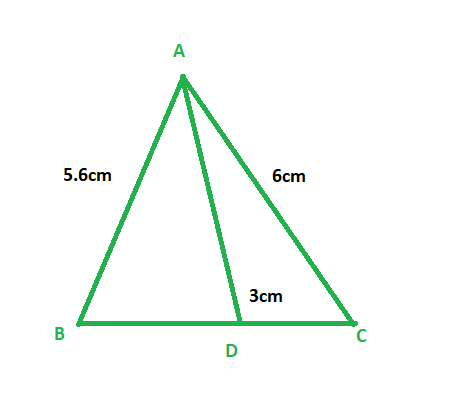Solution:

Given:

Length of side AB = 5.6 cm, BC = 6 cm, and DC = 3 cm

To find: Length of side BC

In Δ ABC, AD is the bisector of  ∠A,  meeting side BC at D

Since, AD is the ∠A bisector

Therefore, we get

⇒ AB/ AC = BD/ DC

5.6/ 6 = BD/ 3

BD = 5.6/ 2 = 2.8cm

And, we know that,

BD = BC – DC

2.8 = BC – 3

2.8 + 3 = BC

∴ BC = 5.8 cm

Therefore, Length of side BC is 5.8 cm

### (vii) If AD = 5.6 cm, BC = 6 cm and BD = 3.2 cm, find AC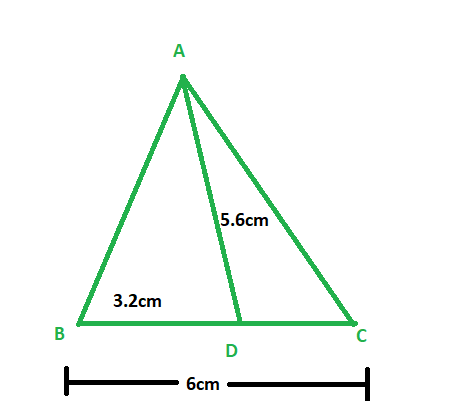Solution:

Given:

Length of side AB = 5.6 cm, BC = 6 cm, and BD = 3.2 cm

To find: Length of side AC

In Δ ABC, AD is the bisector of ∠A, meeting side BC at D

Therefore, we get

⇒ AB/ AC = BD/ DC

5.6/ AC = 3.2/ DC

And, we know that

BD = BC – DC

3.2 = 6 – DC

∴ DC = 2.8 cm

⇒ 5.6/ AC = 3.2/ 2.8

AC = (5.6 × 2.8)/ 3.2

∴ AC = 4.9 cm

Therefore, Length of side AC is 4.9 cm

### (viii) If AB = 10 cm, AC = 6 cm and BC = 12 cm, find BD and DC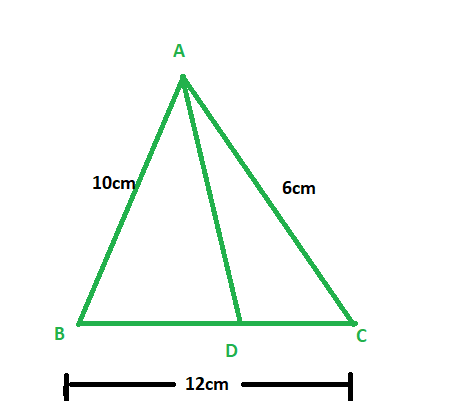Solution:

Given:

Length of side AB = 10 cm, AC = 6 cm, and BC = 12 cm

To find: Length of side BD and DC

In Δ ABC, AD is the ∠A bisector, meeting side BC at D.

Since, AD is bisector of ∠A

Therefore, we get

⇒ AB/ AC = BD/ DC

10/ 6 = BD/ DC                                 – equation 1

And, we also know that

BD = BC – DC = 12 – DC

Let  length of side BD be x,

Then length of side DC will be 12 – x

Now putting values in equation 1, we get

10/ 6 = x/ (12 – x)

5(12 – x) = 3x

60 -5x = 3x

∴ x = 60/8 = 7.5

Hence, DC = 12 – 7.5 = 4.5cm and BD = 7.5 cm

Therefore ,Length of side BD is 7.5 cm and DC is 4.5 cm

### Problem 2: In the figure, AE is the bisector of the exterior ∠CAD meeting BC produced in E. If AB = 10 cm, AC = 6 cm and BC = 12 cm, find CE.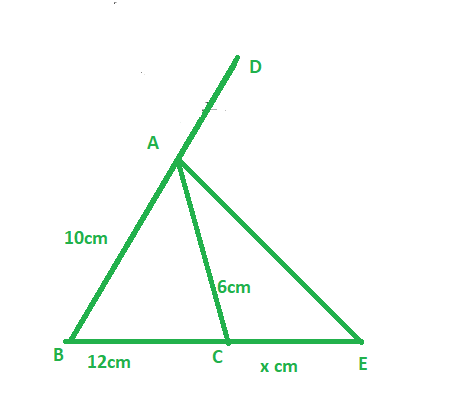Solution:

Given:

Length of side AB = 10 cm, AC = 6 cm and BC = 12 cm

And, AE is the bisector of the exterior ∠CAD

To find: Length of side CE

Since, AE is the bisector of the exterior ∠CAD

Therefore, we get,

BE / CE = AB / AC ‘                               – equation 1

Let length of side CE be x

Therefore, BE = 12+ x

Now, putting this value in equation 1

(12+x)/ x = 10/ 6

6x + 72 = 10x

10x – 6x = 72

4x = 72

∴ x = 18

Since CE = x

Therefore, Length of side CE is 18 cm

### Problem 3 : ΔABC is a triangle such that AB/AC = BD/DC, ∠B = 70o, ∠C = 50o, find ∠BAD.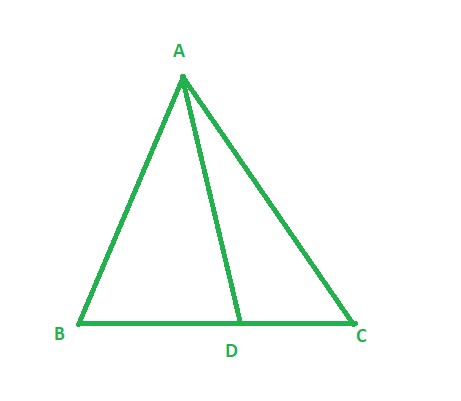Solution:

Given:

Δ ABC such that AB/AC = BD/DC, ∠B = 70o and ∠C = 50o

In Δ ABC,

∠A + ∠B + ∠C = 1800

∠A = 1800 – (70o + 50o)

= 180o – 120o

= 60o

Since, AB/AC = BD/DC

Therefore, AD is the bisector of ∠A

Therefore, ∠BAD = 1/2 (∠A )

Hence, ∠BAD = 60/2 = 30o

### (i) AB = 5 cm, AC = 10 cm, BD = 1.5 cm and CD = 3.5 cm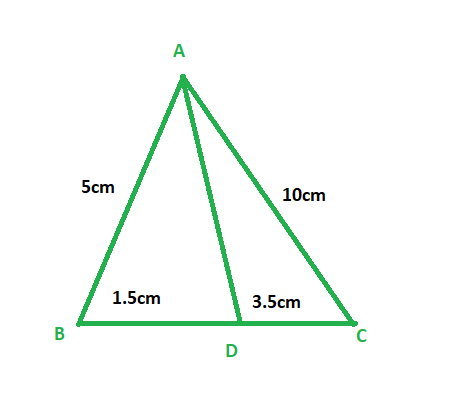Solution:

Given:

Length of side AB = 5 cm, AC = 10 cm, BD = 1.5 cm and CD = 3.5 cm

To check: whether AD is the bisector of ∠A

Now,

AB/AC = 5/10 = 1/2

BD/CD = 1.5/3.5 = 3/7

Therefore,

AB/AC ≠ BD/CD

And since ratio between sides are not proportional

Therefore, AD is not the bisector of ∠A

### (ii) AB = 4 cm, AC = 6 cm, BD = 1.6 cm and CD = 2.4 cm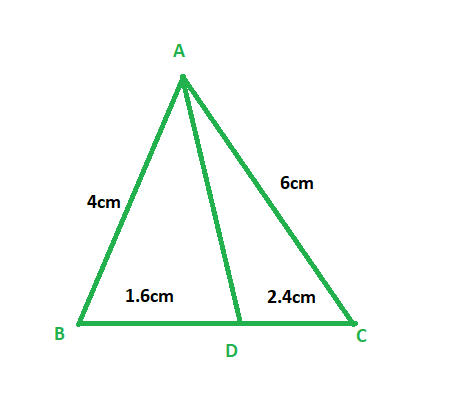Solution:

Given:

Length of side AB = 4 cm, AC = 6 cm, BD = 1.6 cm and CD = 2.4 cm

To check: whether AD is the bisector of ∠A

Now,

AB/AC = 4/6 = 2/3

BD/CD = 1.6/2.4 =2/3

Therefore,

AB/AC = BD/CD

And since ratio between sides are  proportional

Therefore, AD is the bisector of ∠A

### (iii) AB = 8 cm, AC = 24 cm, BD = 6 cm and BC = 24 cm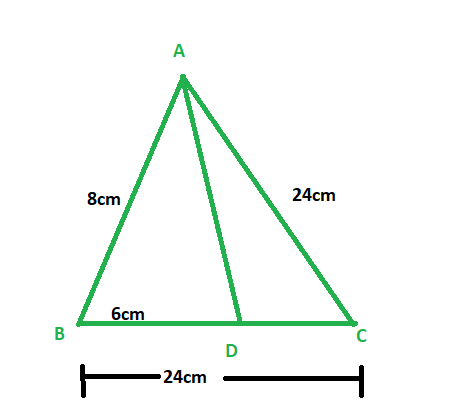Solution:

Given:

Length of side AB = 8 cm, AC = 24 cm, BD = 6 cm and BC = 24 cm

To check : whether AD is the bisector of ∠A

Length of side CD = BC – BD= 24 -6 =18cm

CD = 18cm

Now,

AB/AC = 8/24 = 1/3

BD/CD = 6/18 =1/3

Therefore,

AB/AC = BD/CD

And since ratio between sides are proportional

Therefore, AD is the bisector of ∠A

### (iv) AB = 6 cm, AC = 8 cm, BD = 1.5 cm and CD = 2 cm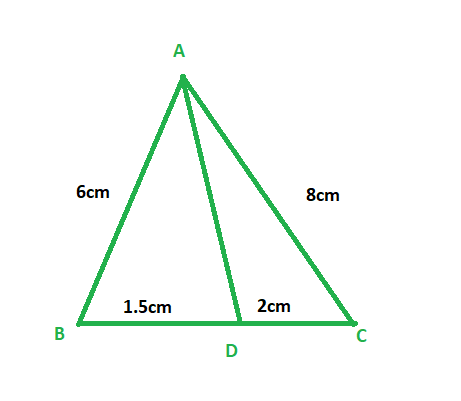Solution:

Given:

Length of side AB = 6 cm, AC = 8 cm, BD = 1.5 cm and CD = 2 cm

To check: whether AD is the bisector of ∠A

Now,

AB/AC = 6/8 = 3/4

BD/CD = 1.5/2 =3/4

Therefore,

AB/AC = BD/CD

And since ratio between sides are proportional

Therefore, AD is the bisector of ∠A

### (v) AB = 5 cm, AC = 12 cm, BD = 2.5 cm and BC = 9 cm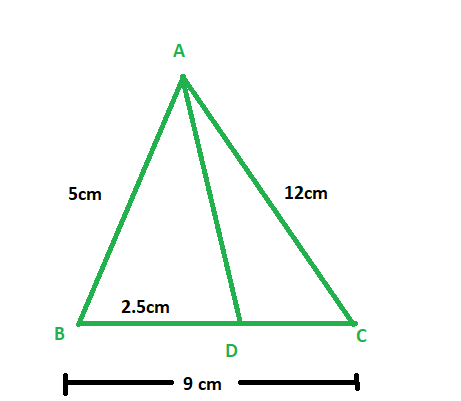Solution:

Given:

Length of side AB = 5 cm, AC = 12 cm, BD = 2.5 cm and BC = 9 cm

To check: whether AD is the bisector of ∠A

Length of side CD = BC – BD= 9 – 2.5 = 6.5cm

CD = 6.5cm

Now,

AB/AC = 5/12 = 5/12

BD/CD = 2.5/6.5 =5/13

Therefore,

AB/AC ≠ BD/CD

And since ratio between sides are not proportional

Therefore, AD is not the bisector of ∠A

### Problem 5: In fig. AD bisects ∠A, AB = 12 cm, AC = 20 cm, and BD = 5 cm, determine CD.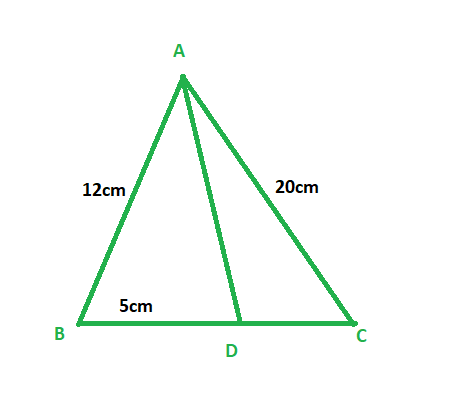Solution:

Given:

Length of side AB = 12 cm, AC = 20 cm, and BD = 5 cm

To find: Length of side CD

Since, AD is the bisector of ∠A

Therefore, we get

AB/AC = BD/CD

12/20 = 5/CD

12 × CD = 20 × 5

CD = 100/12

CD = 8.33 cm

∴ CD = 8.33 cm.

Therefore, Length of side CD is 8.33 cm

### Prove that,  AB/AC = BD/CD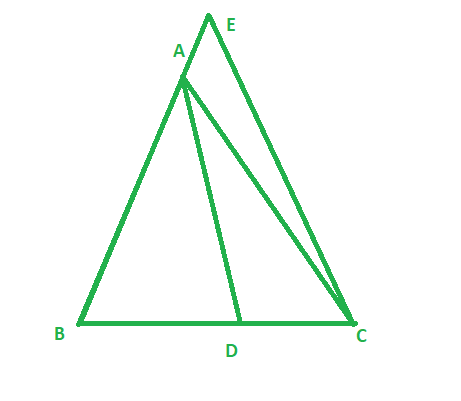Solution:

Given:

∠1 = ∠2

To prove : AB/AC = BD/CD

Construction: Through C , draw CE || BA which meets BA in E on producing the line further

Proof :

Therefore, ∠2 = ∠3        (Alternate angle )

And, ∠1 = ∠4                (Corresponding angle)

And ∠1 = ∠2           (Given)

Therefore, ∠3 = ∠4

Since, sides opposite to equal angles are equal

So, AC = AE              – equation 1

Now, in ΔBCE

So, AD is the bisector of ∠A

Therefore, we get

AB/AE = BD/CD

Since, AC = AE from equation 1

Therefore, AB/AC = BD/CD

Hence proved

### Problem 7 : D and E are the points on sides BC, CA and AB respectively. of a ΔABC  such that AD bisects ∠A, BE bisects ∠B and CF bisects ∠C. If AB = 5 cm, BC = 8 cm, and CA = 4 cm, determine AF, CE, and BD.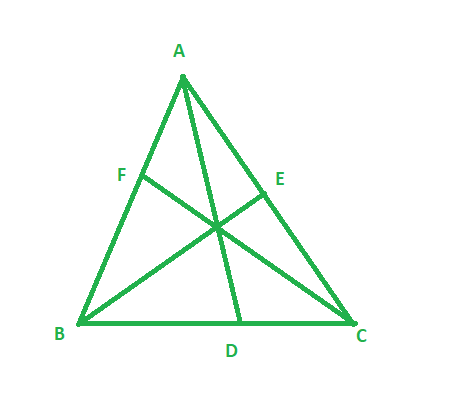Solution:

Given:

Length of side AB = 5 cm, BC = 8cm, and CA = 4 cm

AD bisects  ∠A, BE bisects ∠B and CF bisects ∠C

To find: Length of side AF, CE, and BD

Since, AD is the bisector of ∠A

Therefore, we get,

AB/AC = BD/CD

5/4 = BD/ (BC – BD)                      ( Since CD = BC – BD )

5/4 = BD/ (8 – BD)

40 – 5BD = 4BD

9BD = 40

Therefore, BD = 40/9

Since, BE is the bisector of ∠B

Therefore, we get,

AB/BC = AE/EC

5/8 = (AC – EC)/EC                       ( Since AE = AC – EC )

5/8 = (4 – EC)/EC

5EC = 8(4 – EC)

5EC = 32 -8EC

13EC =32

EC = 32/13

Therefore, EC = 32/13

Now, since, CF is the bisector of ∠C

Therefore, we get,

BC/CA = BF/AF

8/4 = (AB – AF)/AF                                  ( Since BF = AB – AF )

2 = (5 – AF)/AF

2AF = 5 – AF

3AF = 5

AF = 5/3

Therefore, AF = 5/3

So length of BD is 40/9cm, EC is 32/13cm and AF is 5/3 cm

My Personal Notes arrow_drop_up